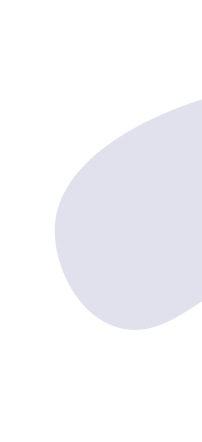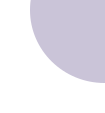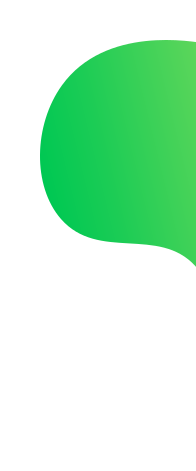## Course packages

The course Mathematics 1 part 1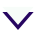Basic course for children from 4 to 6 years old of various levels of development and knowledge. By the end of the course of study, the main result should be the formation of children's interest in knowledge, the development of their attention, memory, speech, and mental operations.

Kids will:
• know, distinguish and correlate the basic colors and color shades of objects;
• know the sequence of the first ten numbers and the place of each number in natural order;
• be able to distinguish between numerical and ordinal counting within ten;
• be able to name the previous and the next number for each number, continue counting both forward and backward from any given number;
• be able to compare the numbers standing next to each other (within ten);
• be able to distinguish and read printed numbers, correlate them with the corresponding set of objects, specified using numerical figures and object pictures;
• know the composition of numbers (numbers of the first ten);
• be able to distinguish and apply the signs of mathematical operations (addition, subtraction, equality, more or less);
• be able to solve examples (addition and subtraction) on object material, fingers;
• be able to make simple examples on visual material;
• be able to fill in a brief condition of the problem and ask questions to find the unknown in the problem;
• understand and be able to solve simple problems using money - "A trip to the store" (within 10);
• be able to identify and compare features of different objects and phenomena using a variety of methods of examination;
• be able to recognize flat geometric elements and figures: point, straight line, curved and broken line, ray, segment, angle, forms of angles, circle, triangle, quadrilateral (square, rectangle); volumetric geometric figures: ball, cube, prism, pyramid, cone, cylinder;
• be able to orient in space (up, down, in front, behind, in front, behind, between, next to, left, right);
• understand the relativity of spatial orientations (higher than, lower than, to the left of, to the right of, above, below, closer than, further than, inside);
• Know the sequence of parts of the day, days of the week, seasons, months of the year;
• understand and apply temporal concepts: earlier - later, now, after - before, fast - slow;
• be able to solve non-standard problems of logical content, to do exercises on the development of attention and memory;
• be able to concentrate on the object under study;
• understand the adult's verbal instructions and act in accordance with them;

Ребёнок будет уметь:
• несколькими методами выделять и сравнивать признаки различных предметов;
• распознавать и называть плоские и объёмные фигуры;
• ориентироваться в пространстве (высоко/низко, слева/справа и т.д.) и понимать пространственные отношения (выше/ниже чем, рядом, перед за и т.д.);
• концентрироваться на изучаемом объекте;
• решать нестандартные логические задачи.
Classes:
40-60
Duration:
7 months
Ages:
from 4 to 6
The course Mathematics 1 part 2This course continuous the course Math 1. For kids from 5 to 7 years old. Suitable for kids who are able to count and solve examples within 10, know and distinguish basic spatial, temporal, and geometric concepts. The course program includes the study of numbers and counting within twenty.

Kids will
• know the sequence of numbers in the second ten and the place of each number in natural order;
• be able to distinguish between numerical and ordinal counting within twenty;
• be able to name the previous and the next number for each number, continue counting both forward and backward from any given number;
• be able to compare the numbers standing next to each other (within twenty);
• know the composition of numbers (numbers of the second ten);
• be able to solve examples (addition and subtraction) on object material, without going through a digit;
• be able to make simple examples on visual material;
• be able to fill in a brief condition of the problem and ask questions to find the unknown in the problem;
• understand and be able to solve simple problems using money - "A trip to the store" (within 20);
• be able to measure flat geometric figures using a ruler: segment, sides of a triangle, quadrangle (square, rectangle), distance between objects;
• understand and distinguish between mathematical concepts: area, volume, mass;
• know and set the time on mechanical and electronic watches;
• understand and correlate the time on the watches to the part of the day;
• be able to solve non-standard problems of logical content, to do exercises on the development of attention and memory;
Classes:
40-60
Duration:
7 months
Ages:
from 5 to 7
The course Mathematics 2This course continuous the course Math 1. For kids from 6 to 8 years old. Suitable for kids who are fluent in counting and solving within 20 without going through a digit, use a ruler to measure and have an idea of time, can set the time on a clock.

Kids will:
• know the sequence of tens of the first hundred numbers and the place of each ten in natural order;
• be able to solve examples (addition and subtraction) on object material, without going through a digit;
• be able to solve examples (multiplication and division) on object material (within 20);
• be able to draw flat geometric figures using a ruler and a pencil: straight line, ray, segment, sides of a triangle, quadrangle (square, rectangle);
• be able to solve examples and problems to find time intervals;
• be able to find and compare areas of geometric figures, volume and mass of bodies;
• be able to solve non-standard problems of logical content, to do exercises on the development of attention and memory;
Classes:
80-100
Duration:
12 months
Ages:
from 6 to 8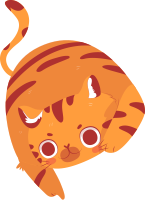## Try a free trial class

Fill in the form below and we’ll contact you to discuss your learning options

Phone
By clicking “Try it for free”, you agree to our Privacy policy and accept Terms and Conditions
Please fill in a valid value for all required fields!